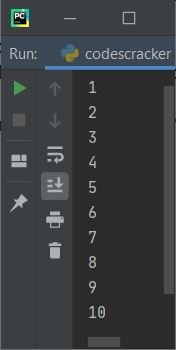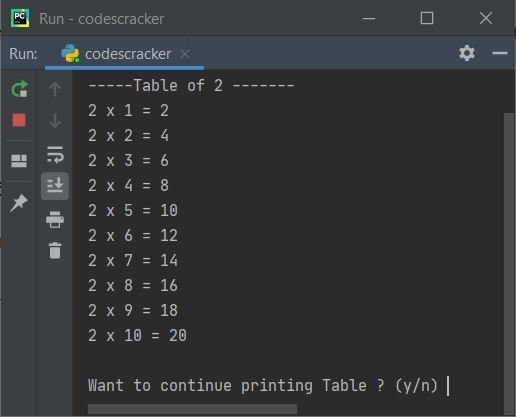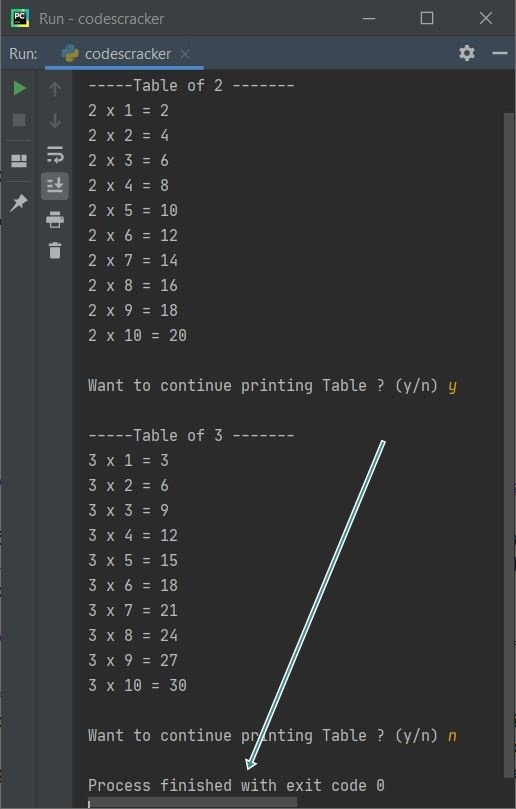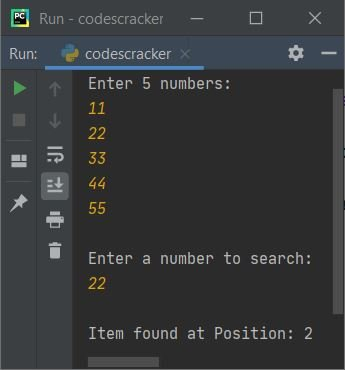# break statement in Python

This tutorial will go over the second most commonly used conditional statement in Python, "break." Here I've provided you with all the details about the "break" keyword or statement, along with some simple examples. This article deals with:

## What is a "break" statement?

The "break" statement in Python is used to exit a loop. In other words, we use the "break" keyword to terminate the remaining execution of the whole or complete loop in its indentation. Don't worry about the definition; you'll get to know everything about it after understanding the examples given below.

## Syntax of the break statement

The syntax to use the "break" keyword is only the keyword itself. Therefore, its syntax is:

`break`

That's it. We do not need anything other than the keyword itself.

## Examples of a break statement

Now let's have a look at some of the examples of the "break" keyword or statement. Let's start with the "break" statement in the while loop example.

### break statement in the while loop

This program contains a break inside the while loop.

```count = 0
while True:
count = count+1
if count>10:
break
print(count)```

This program produces the output shown in the snapshot given below:As you can see from the above output, when the variable "count" becomes greater than 10, That is, when the value of "count" becomes 11, then the condition "count>10" evaluates to be "True," so the program flow goes inside the if's body and the statement "break" gets executed, which leaves the loop for further execution or simply terminates the remaining execution of the "while" loop.

Let's take another example of the "break" keyword or statement that helps a lot in understanding the benefit of using "break" in Python:

```num = 2
while True:
mul = 1
print("\n-----Table of", num, "-------")
while mul<=10:
print(num, "x", mul, "=", num*mul)
mul += 1
print("\nWant to continue printing Table ? (y/n) ", end="")
confirm = input()
if confirm == 'n':
break
num += 1```

This is the initial output produced by the above program:After printing table 2, the program prompts the user to choose whether to print the next table or skip or stop printing that table. To confirm, enter y to print the next table; otherwise, enter n to stop. For example, the output given below shows what is produced after providing y as input:Since the condition of the while loop is given as true, that always evaluates to true, and the execution of the loop never ends until and unless you use the break keyword. And to do this, we have to enter n as input, so that the condition confirm=="n" evaluates to be True and the program flow goes inside the if's body and executes the break keyword, which helps in exiting or leaving the loop, as shown in the sample run given below:### break statement in the nested while loop

This program uses the break keyword in a while loop present inside another while loop:

```count = 0
while count<10:
count = count+1
while count<5:
if count==3:
break
count = count+1
print(count)```

This program produces the following output:The following is how the above program is run:

• First, "0" gets initialized to "count."
• Now the condition "count<10" or "0<10" evaluates to true.
• Therefore, program flow goes inside the body of the "while" loop.
• Inside the body of the loop, the first statement, which is "count = count+1," gets executed.
• Therefore, "count+1" or "0+1" or "1" gets initialized to "count."
• Now the condition "count<5" or "1<5" evaluates to true.
• Therefore, program flow goes inside the body of the loop (the inner "while" loop).
• Furthermore, the condition "count == 3" or "1 == 3" evaluates to False.
• Therefore, program flow does not go inside the body of the "if" block.
• The statement "count = count+1" (inside the inner loop) gets executed.
• That is, "count+1" or "1+1" or "2" gets initialized to "count."
• All the statements of the inner loop get executed.
• The program flow again evaluates the condition.
• The condition "count<5" gets evaluated again.
• This time, "count<5" or "2<5" evaluates to true.
• Therefore, program flow again goes inside the loop.
• And the condition "count == 3" or "2 == 3" again evaluates to false.
• Now "count+1" or "2+1" or "3" gets initialized to "count."
• Program flow again evaluates the condition of the inner loop. This process continues until the condition is evaluated as false.
• That is, the condition "count<5" or "3<5" again evaluates to true.
• Therefore, program flow again goes inside this loop.
• But this time, the condition of "if,"  that is, "count == 3" or "3 == 3," evaluates to true.
• Therefore, program flow goes inside this "if's" body.
• And using the "break" keyword, we've left the loop (the inner "while" loop).
• That is, using "break,"  the remaining execution of its nearest parent loop gets terminated or stopped.
• After exiting the loop, using the "print()" statement, the value of "count" gets printed. That is "3."
• As all the statements of the outer "while" loop get executed
• Therefore, its condition again gets evaluated. This process continues until the condition is evaluated as false.
• That is, the condition "count<10" or "3<10" evaluates to true again.
• Therefore, program flow again goes inside the loop, and "count+1" or "3+1" or "4" gets initialized to "count."
• And the condition of the inner loop, "count<5", gets evaluated.
• That is, the condition "count<5" or "4<5" evaluates to true.
• Therefore, program flow inside this inner loop
• Inside this loop, the condition (of "if") "count==3" or "4==3" evaluates to false.
• Therefore, program flow does not go inside its body. The value of "count" is incremented.
• That is, the value of "count" now equals 5.
• And this time the condition of the inner loop, which is "count<5" or "5<5", evaluates to false.
• Therefore, the inner "while" loop's execution stops now. Because the condition will now evaluate to false every time.
• Now, the values 5 to 10 are printed one by one on the output.
• That is, the values "1," "2," and "4" do not get printed.

### break statement in the for loop

The program given below uses a break statement in a for loop:

```for i in range(10000000):
if i==5:
break
print(i)```

Here is its sample output:As you can see, using the break statement, I've stopped the remaining execution of the loop when the value of i becomes equal to 5.

Let's take another example that shows you the most important use of the break statement:

```nums = list()
print("Enter 5 numbers: ")
for i in range(5):
nums.append(int(input()))

print("\nEnter a number to search: ")
n = int(input())

for i in range(5):
if n==nums[i]:
print("\nItem found at Position:", i+1)
break```

Here is its sample run with user inputs: 11, 22, 33, 44, 55 as five numbers, and 22 as the number to search for:Note: The break keyword helps a lot to prevent the unwanted execution of codes. As you can see from the above program, when our work was completed, i.e. when the element was discovered, then we don't need to execute or search for any further elements. Therefore, using the break keyword, we simply exit from the loop. That's it.

Python Online Test

« Previous Tutorial Next Tutorial »